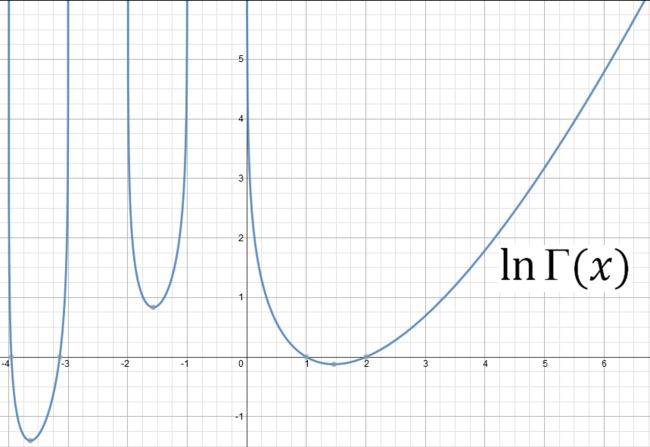# Log o' Gamma

Calculus Level 5$\large \int_{2}^{3} \ln(\Gamma(x))\, dx$ If the result of the integral above can be expressed in the form $\large \frac{a}{c}\ln{c}+\frac{b}{c}\ln{\pi}-c$ where $\large a,b$ and $\large c$ are positive coprime integers. Find the value of $\large a+b+c$.

Note that $\Gamma(x)$ is the Gamma Function.

×